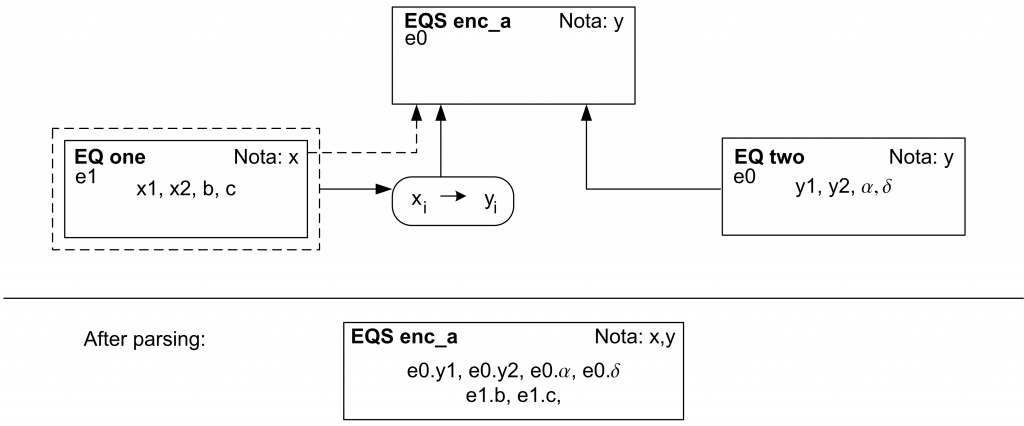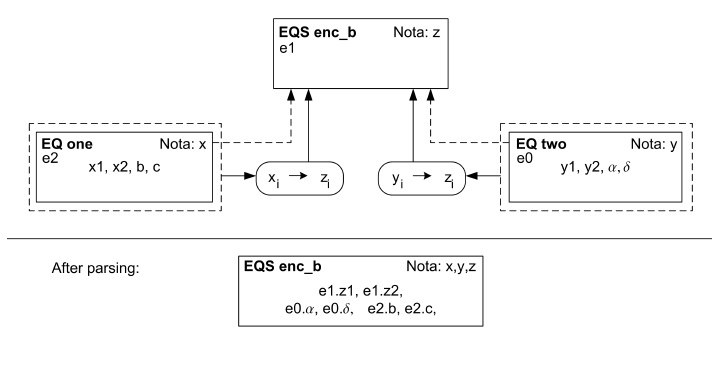# Connection Techniques II – Naming Policy ‘encapsulate’

Stefan Kuntsche, Sanam Sabeti Adibpour, Gregor Tolksdorf

It is assumed that you have already worked through the first section of connectors.

##### Rules

The basic contract of the naming policy ‘encapsulate’ is as follows:

• Basic rule: If an equation or equation system (sub element) is added to a super equation system (super element), the sub element’s variables will get an own namespace.
• Additional rule using a connector: If a sub element is added to a super element by using a connector, only those variable names of the sub element that are translated by the connector will get the super element’s namespace.
• Result: When considering the sub element, only the variables translated by a connector have a top level naming according to the super notation.
##### ‘Encapsulate’ case a: Two notations involved.

In this example case one connected equation uses a different notation. Only one (generic) variable needs to be translated as the other variables do not appear in the super equation system (not even within the naming convention of the super notation).

Equation System ‘encaps a’$y_{j=1} + \beta \cdot y_{j=2} = \gamma$$\delta \cdot (y_{j=2})^{2} = -\alpha + y_{j=1}$

Notation y

Base Names:

[table sort=”desc,asc”]
Name, Description$y$,values$\alpha$,parameter 1$\beta$,parameter 2$\gamma$,parameter 3$\delta$,parameter 4
[/table]

Indices:

[table sort=”desc,asc”]
Name, Description$j$,value index-max val: Nj
[/table]

Collaboration

In this example, an equation is taken from another project. To avoid reimplementation the existing equation using ‘Notation x’ is employed here.

Equation one$x_{i=1} + b \cdot x_{i=2} = c$

Notation x

Base Names:

[table sort=”desc,asc”]
Name,Description$x$,values$a$,parameter 1$b$,parameter 2$c$,parameter 3$d$,parameter 4
[/table]

Indices:

[table sort=”desc,asc”]
Name,Description$i$,value index-max val: Ni
[/table]
Connector selective one

• Subnotation: Notation x
• Supernotation: Notation y
• Value list:$x_{i}\rightarrow y_{j}$Figure 1: Graph of the variable interpretation

After the parsing process the equations have the following appearance:$e0.y_{j=1} + e1.b \cdot e0.y_{j=2} = e1.c$$e0.\delta \cdot (e0.y_{j=2})^{2} = -e0.\alpha + e0.y_{j=1}$

Alternatively, the user can decide to view the equations without namespaces:$y_{j=1} + b \cdot y_{j=2} = c$$\delta \cdot (y_{j=2})^{2} = -\alpha + y_{j=1}$

This view, however, might be confusing as names of different variables can appear identical.

Problem description

Design variables with values:$e0.\alpha = 1$$e0.\delta = 2$$e1.b = 1$$e1.c = 3$

Iteration variables with guess values:$e0.y_{j=1} = 1$$e0.y_{j=2} = 1$

Iteration variables with result values:$e0.y_{j=1} = 2.22$$e0.y_{j=2} = 0.78$

Re-use of model elements This section is based on previous sections. Usually you should have created many of the needed model parts. We will try to use as much of the existing parts as possible.

Creating the connector

• In the Editor bar click on Connector tab and open ‘Connector one’ that you have created in Connection techniques I and save it as a new one named ‘Connector selective one’.
• Activate tab Edit Matching.
• In the table Matching select the row for b and$\beta$.
• Press [Break]. The symbols b and$\beta$ should have been removed from the Matching table and be present in the tables Sub Notation and Super Notation respectively.
• Remove all matchings except for$x_{i}\rightarrow y_{i}$ from the Matching table as described above.
• In the Test Connector tab load ‘Equation one’ created in Connection techniques I. If everything went well, only the instances of$x{i}$ should be matched to instances of$y_{i}$.
• Save the connector.

Creating the equation system

• In the Equation System Editor specify ‘Notation y’ as the equation system’s notation.
• Press [Add] in the lower right corner, which brings up the Edit Connection to Element dialog.
• In the dialog press [Change] for the Conn. Elem. field.
• Select ‘Equation one’ created in Connection techniques I.
• In the Edit Connection to Element dialog use the drop down list box next to Naming policy and change the value to encapsulate.
• In the Advanced section tick the field Use connector and press [Change] next to the corresponding field.
• Select ‘Connector selective one’, which you created above in this tutorial.
• Press [Submit].
• Add ‘Equation two’ created in Connection techniques I using the naming policy ‘integrated’ and without loading any connector.
• Save the equation system.

Evaluating the equation system

• Activate the Evaluation Editor and load the equation system ‘encaps_a’ you just created.
• In the Indexing tab specify 2 as a maximum value for the index i.
• Now have a look at the equation system in the Instance tab.
• Press [Name Spaces], which toggles the views between the view without the namespaces and the view including the namespaces.
• On the tab Info you find the specification of the two namespaces involved.
• Solve the modeling task as usual entering the rest of the information given under Problem description above.
##### ‘Encapsulate’ case b: Three notations involved.

In this example the connected equations have different notations. They have only one common (generic) variable$z_{i}=y_{i}=x_{i}$. Using the naming policy encapsulate makes it possible to specify only the translation of this common variable and to assure at the same time that all other variables are not matched by accident.

Equation System ‘encaps b’$z_{k=1} + B \cdot z_{k=2} = C$$D \cdot (z_{k=2})^{2} = -A + z_{k=1}$

Notation z

Base Names:

[table sort=”desc,asc”]
Name,Description$z$,values$A$,parameter 1$B$,parameter 2$C$,parameter 3$D$,parameter 4
[/table]

Indices:

[table sort=”desc,asc”]
Name,Description$k$,value index-max val: Nk
[/table]

Collaboration

In this example, equations (1) and (2) are taken from other projects. To avoid reimplementation, existing equations are used here. The only translation is made to$z_{i}$ from$x_{i}$ and$y_{i}$ respectively.

Equation one$x_{i=1} + b \cdot x_{i=2} = c$

Notation x

Base Names:
[table sort=”desc,asc”]
Name,Description$x$,values$a$,parameter 1$b$,parameter 2$c$,parameter 3$d$,parameter 4
[/table]

Indices:

[table sort=”desc,asc”]
Name,Description$i$,value index
[/table]

Connector x-z

• Subnotation: Notation x
• Supernotation: Notation z
• Value list:$x_{i}\rightarrow z_{k}$

Equation two$y_{j=1} + \beta \cdot y_{j=2} = \gamma$

Notation y

Base Names:

[table sort=”desc,asc”]
Name,Description$y$,values$\alpha$,parameter 1$\beta$,parameter 2$\gamma$,parameter 3$\delta$,parameter 4
[/table]

Indices:

[table sort=”desc,asc”]
Name,Description$j$,value index-max val: Nj
[/table]

Connector y-z

• Subnotation: Notation y
• Supernotation: Notation z
• Value list:$y_{j}\rightarrow z_{k}$Figure 2: Graph of the variable interpretation

View of the equation system with namespaces:$e1.z_{k=1} + e2.b \cdot e1.z_{k=2} = e2.c$$e0.\delta \cdot (e1.z_{k=2})^{2} = -e0.\alpha + e1.z_{k=1}$

View of the the equation system without namespaces:$z_{k=1} + b \cdot z_{k=2} = c$$\delta \cdot (z_{k=2})^{2} = -\alpha + z_{k=1}$

Problem description

Design Variables with values:$e0.\alpha = 1.0$$e0.\delta = 2.3$$e2.b = 3.5$$e2.c = 4.5$

Iteration Variables with guess values:$e1.z_{k=1} = 1$$e1.z_{k=2} = 1$

Iteration Variables with result values:$e1.z_{k=1} = 2.27$$e1.z_{k=2} = 0.74$

##### Creating the connectors and the equation system
• Create the connectors ‘Connector x-z’ and ‘Connector y-z’.
• Create the equation system ‘encaps b’ using ‘Notation z’.
• Add ‘Equation one’ to ‘encaps b’ specifying the naming policy as ‘encapsulate’ and choosing ‘Connector x-z’.
• Add ‘Equation two’ to ‘encaps b’ as ‘encapsulate’ and choose ‘Connector y-z’.
##### Evaluating the equation system
• Load equation system ‘encaps b’ into the Evaluation Editor and specify the maximum value of the index$k$ to 2.
• Look again at the instance of the equation system in the Instance tab and toggle between the different views using the [Name Spaces] button.
• In the Info tab the three name spaces are specified.
• In the Variable Specification tab select the variable$z_{k=1}$ and use the buttons [T], [$\downarrow$] and [$\uparrow$] to navigate between the different namings of this variable.
• Enter the missing pieces of the problem description and solve the problem.